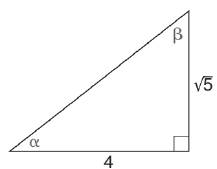Chapter 11.3, Problem 25E### Elementary Geometry for College St...

6th Edition
Daniel C. Alexander + 1 other
ISBN: 9781285195698

#### Solutions

Chapter
Section### Elementary Geometry for College St...

6th Edition
Daniel C. Alexander + 1 other
ISBN: 9781285195698
Textbook Problem
1 views

# In Exercises 21 to 26, use the sine, cosine, or tangent ratio to find the indicated angle measures to the nearest degree.To determine

To find:

The indicated angle measures to the nearest degree using the sine, cosine or tangent ratio.

Explanation

Given:

The triangle with indicated angles is shown below.

Definition used:

The trigonometric ratios:

Calculation:

Now consider the angle α.

From the figure,

tanα=54

α=tan1(54)

Let us use Ti-83 calculator to find the value of α.

Press ON in Ti-83 calculator.

Since we need to find the value of degree, we need to change the mode to degrees.

Press MODE button and use arrow keys to select the “Degree” mode.

Now press the following buttons in the same sequence.

2ndTAN2ndx2(5)÷4

Then press ENTER

### Still sussing out bartleby?

Check out a sample textbook solution.

See a sample solution

#### The Solution to Your Study Problems

Bartleby provides explanations to thousands of textbook problems written by our experts, many with advanced degrees!

Get Started

#### Find more solutions based on key concepts